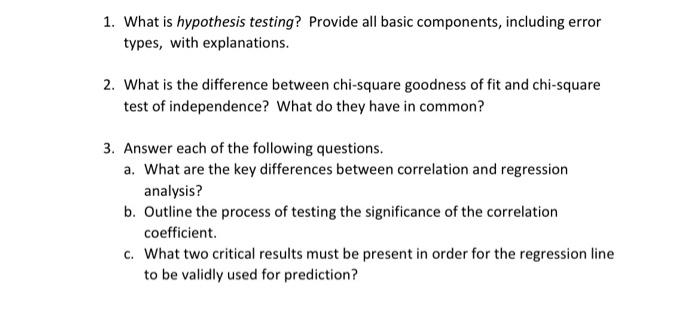# Question 1. What is hypothesis testing? Provide all basic components, including error types, with explanations. 2. What is the difference between chi-square goodness of fit and chi-square test of independence? What do they have in common? 3. Answer each of the following questions. a. What are the key differences between correlation and regression analysis? b. Outline the process of testing the significance of the correlation coefficient c. What two critical results must be present in order for the regression line to be validly used for prediction?AGSHA6 The Asker · Probability and StatisticsTranscribed Image Text: 1. What is hypothesis testing? Provide all basic components, including error types, with explanations. 2. What is the difference between chi-square goodness of fit and chi-square test of independence? What do they have in common? 3. Answer each of the following questions. a. What are the key differences between correlation and regression analysis? b. Outline the process of testing the significance of the correlation coefficient c. What two critical results must be present in order for the regression line to be validly used for prediction?
More
Transcribed Image Text: 1. What is hypothesis testing? Provide all basic components, including error types, with explanations. 2. What is the difference between chi-square goodness of fit and chi-square test of independence? What do they have in common? 3. Answer each of the following questions. a. What are the key differences between correlation and regression analysis? b. Outline the process of testing the significance of the correlation coefficient c. What two critical results must be present in order for the regression line to be validly used for prediction?